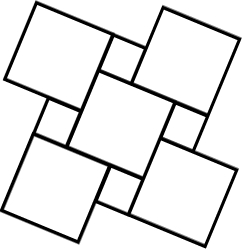# Unpainted 14513

The abcd square is composed of 36 small squares. Six of them are painted. How many small squares do we still need to color so that a quarter of the area of ​​the abcd square remains unpainted?

n =  21

### Step-by-step explanation:Did you find an error or inaccuracy? Feel free to write us. Thank you!

Tips for related online calculators
Need help calculating sum, simplifying, or multiplying fractions? Try our fraction calculator.
Do you have a linear equation or system of equations and looking for its solution? Or do you have a quadratic equation?

#### Grade of the word problem:

We encourage you to watch this tutorial video on this math problem: